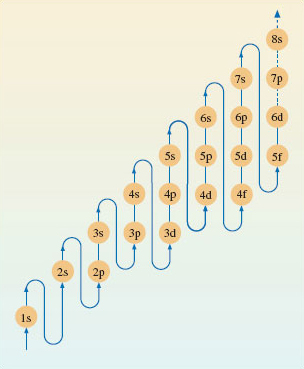Discovering chemistry

Start this free course now. Just create an account and sign in. Enrol and complete the course for a free statement of participation or digital badge if available.

Free course

# 1.8.1 Electronic configurations of multi-electron atoms.

Electronic configurations can be assigned to nearly all atoms using the diagram shown in Figure 7.Figure 7 A pathway showing the order in which the sub-shells should be filled when writing out the electronic configurations of atoms

Consider fluorine, this has an atomic number of 9, which means there are 9 electrons to fit into the subshells shown in Figure 7.

Bearing in mind the maximum number of electrons a sub-shell can hold (shown in Figure 6), the electrons in a fluorine atom will be arranged as follows:

Two electrons will be in the lowest energy 1s subshell.

Two electrons will be in the higher energy 2s subshell.

This leaves another five electrons which occupy the 2p subshell.

So using the notation introduced earlier, the electronic configuration for fluorine may be written:

1s2 2s2 2p5.

Now have a go at the following examples (remember you’ll need to refer back to Figure 7 ).

What are the electronic configurations of lithium (atomic number 3), silicon (atomic number 14) and potassium (atomic number 19)?

Lithium: 1s2 2s1

Silicon: 1s2 2s2 2p6 3s2 3p2

Potassium: 1s2 2s2 2p6 3s2 3p6 4s1

You probably noticed a quirk in the expected order of energy level filling which became apparent when you got to potassium. The 4s sub-shell is at lower energy than the 3d subshell. So this means the 4s shell fills with electrons before the 3d.

Try the following example which also highlights this point.

What is the electronic configuration of titanium (atomic number 22)?

1s2 2s2 2p6 3s2 3p6 4s2 3d2

For a bit more practice have a go at the following examples:

What are the electronic configurations of the following elements (these are the ones shown in Figure 1)?

(a) aluminium (atomic number 13), (b) sodium (atomic number 11), and copper (atomic number 29).

Al: 1s2 2s2 2p6 3s2 3p1

Na: 1s2 2s2 2p6 3s1

Cu: 1s2 2s2 2p6 3s2 3p6 4s2 3d9

DC_1